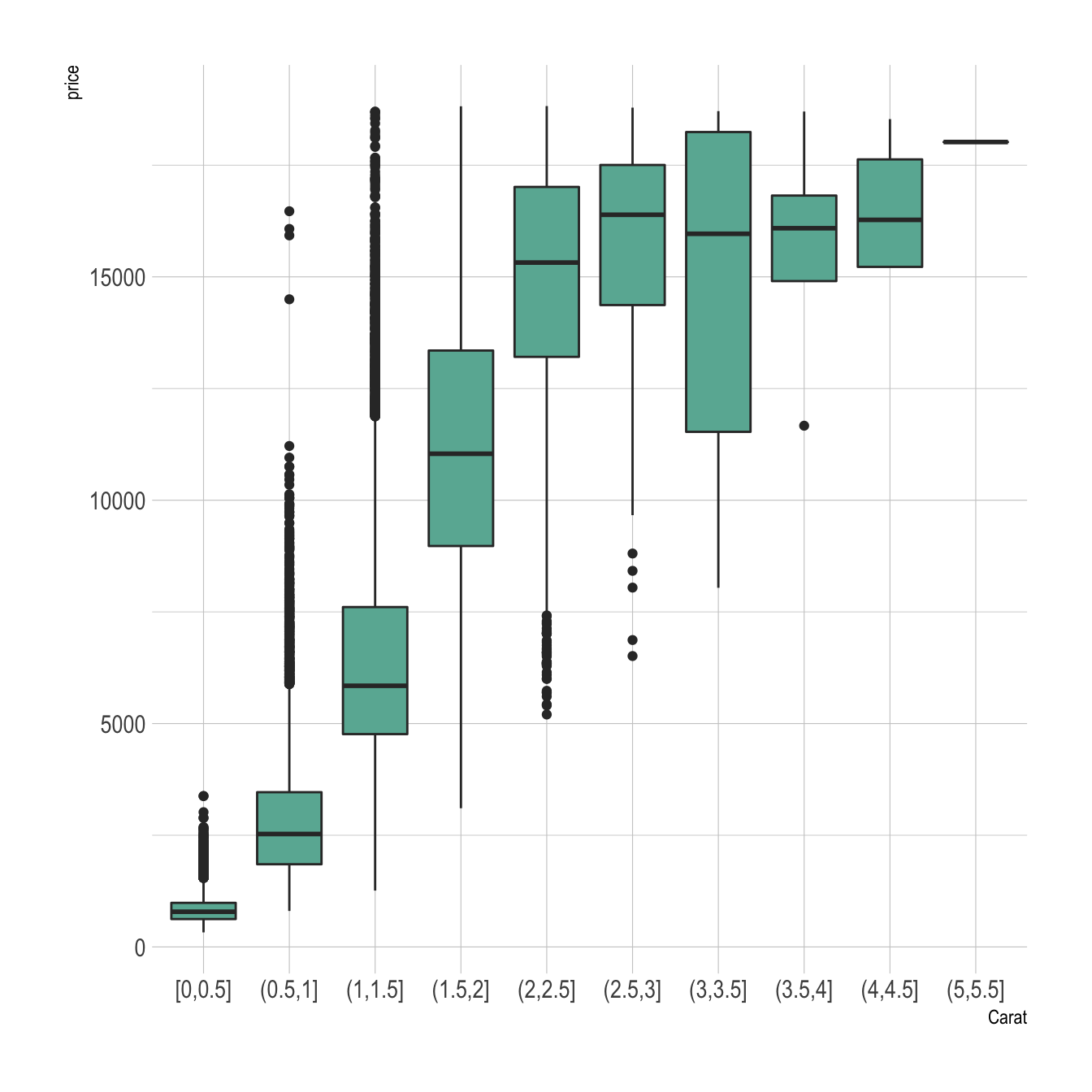# ggplot2 boxplot from continuous variable

A boxplot summarizes the distribution of a continuous variable. This post explains how to build a boxplot with ggplot2 where categories are actually bins of a numeric variable. It is sometimes useful to study the relationship between 2 nnumeric variables.

Let’s say we want to study the relationship between 2 numeric variables. It is possible to cut on of them in different bins, and to use the created groups to build a boxplot.

Here, the numeric variable called `carat` from the `diamonds` dataset in cut in 0.5 length bins thanks to the `cut_width` function. Then, we just need to provide the newly created variable to the X axis of `ggplot2`.

``````# library
library(ggplot2)
library(dplyr)
library(hrbrthemes)

p <- diamonds %>%

# Add a new column called 'bin': cut the initial 'carat' in bins
mutate( bin=cut_width(carat, width=0.5, boundary=0) ) %>%

# plot
ggplot( aes(x=bin, y=price) ) +
geom_boxplot(fill="#69b3a2") +
theme_ipsum() +
xlab("Carat")``````Related chart types

## Contact

This document is a work by Yan Holtz. Any feedback is highly encouraged. You can fill an issue on Github, drop me a message on Twitter, or send an email pasting yan.holtz.data with gmail.com.